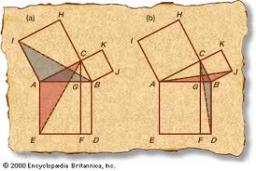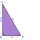# Euclid3

Calculate height and sides of the right triangle, if one leg is a = 81 cm and section of hypotenuse adjacent to the second leg cb = 39 cm.

a =  81 cm
b =  63.3 cm
c =  102.8 cm
h =  49.9 cm

### Step-by-step explanation:Did you find an error or inaccuracy? Feel free to write us. Thank you!Tips to related online calculators
Looking for help with calculating roots of a quadratic equation?
Do you want to convert length units?

#### You need to know the following knowledge to solve this word math problem:

We encourage you to watch this tutorial video on this math problem:

## Related math problems and questions:

• Euclid theoremsCalculate the sides of a right triangle if leg a = 6 cm, and a section of the hypotenuse, which is located adjacent to the second leg b is 5cm.
• Triangle KLMIn the rectangular triangle KLM, where is hypotenuse m (sketch it!). Find the length of the leg k and the height of triangle h if the hypotenuse's segments are known MK = 5cm and ml = 15 cm.
• Euclid 5Calculate the length of remain sides of a right triangle ABC if a = 7 cm and height vc = 5 cm.
• Hypotenuse and heightIn a right triangle is length of the hypotenuse c = 56 cm and height hc = 4 cm. Determine the length of both trangle legs.
• RT leg and perimeterCalculate the length of the sides of a right triangle ABC with hypotenuse c when the length of a leg a= 84 and perimeter of the triangle o = 269.
• Right ΔA right triangle has the length of one leg 11 cm and the hypotenuse 61 cm size. Calculate the height of the triangle.
• Sides of the triangleCalculate triangle sides where its area is S = 84 cm2 and a = x, b = x + 1, xc = x + 2
• Without Euclid lawsRight triangle ABC with right angle at the C has a=14 and hypotenuse c=26. Calculate the height h of this triangle without the use of Euclidean laws.
• Euclid1Right triangle has hypotenuse c = 27 cm. How large sections cuts height hc=3 cm on the hypotenuse c?
• Right triangleLegs of the right triangle are in the ratio a:b = 2:8. The hypotenuse has a length of 87 cm. Calculate the perimeter and area of the triangle.
• RT perimeterThe leg of the rectangular triangle is 7 cm shorter than the second leg and 8 cm shorter than the hypotenuse. Calculate the triangle circumference.
• One legOne leg of a right triangle is 1 feet longer than the other leg. The hypotenuse is 5 feet. Find the lengths of the three sides of the triangle.
• Right triangle AlefThe obvod of a right triangle is 84 cm, and the hypotenuse is 37 cm long. Determine the lengths of the legs.
• Hypotenuse - RTA triangle has a hypotenuse of 55 and an altitude to the hypotenuse of 33. What is the area of the triangle?
• Sides of right angled triangleOne leg is 1 m shorter than the hypotenuse, and the second leg is 2 m shorter than the hypotenuse. Find the lengths of all sides of the right-angled triangle.
• RT - hypotenuse and altitudeRight triangle BTG has hypotenuse g=117 m and altitude to g is 54 m. How long are hypotenuse segments?
• Right triangle eq2Hypotenuse of a right triangle is 9 cm longer than one leg and 8 cm longer than the second leg. Determine the circumference and area of a triangle.